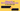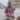# Object.is() vs Strict Equality Operator in JavaScript

Posted May 31, 2020Start discussion

The triple equality operator `===` checks strictly whether 2 values are the same:

``````1 === 1;    // => true
1 === '1';  // => false
1 === true; // => false``````

However, ES2015 specification brings `Object.is()`, which behaves almost same way as strict equality operator:

``````Object.is(1, 1);    // => true
Object.is(1, '1');  // => false
Object.is(1, true); // => false``````

The main question is: when would you use Object.is() instead of a strict equality check? Let’s find out.

## 1. Strick equality check operator

To begin with, let’s refresh quickly how the strict equality operator works.

The strict equality check operator evaluates to `true` when both values are of the same type and hold the same value.

For example, the following primive values are equal because they’re the same type and have the same value:

``````1         === 1;         // => true
'abc'     === 'abc';     // => true
true      === true;      // => true
null      === null;      // => true
undefined === undefined; // => true``````

The strict equality operator doesn’t perform type coersion of operators. Even if operators hold reasonable same values, but nevertheless of different types, they aren’t strictly equal:

``````1    === '1';       // => false
1    === true;      // => false
null === undefined; // => false``````

When performing the strict equality check on objects, an object is strictly equal only to itself:

``````const myObject = { prop: 'Value' };
myObject === myObject;  // => true``````

Even if 2 objects have exactly the same properties and values, they’re different values:

``````const myObject1 = { prop: 'Value' };
const myObject2 = { prop: 'Value' };
myObject1 === myObject2; // => false``````

The above comparison scenarios work exactly the same in `Object.is(valueA, valueB)`.

The difference between strict equality check and `Object.is()` lies in how `NaN` and how negative zero `-0` are treated.

Firstly, `NaN` (Not A Number) isn’t strictly equal to any other value, even with another `NaN`:

``````NaN === NaN; // => false
NaN === 1;   // => false``````

Secondly, the strict equality operator doesn’t distinguish `-0` from `+0`:

``-0 === +0; // => true``

The strict equality operator uses the Strict Equality Comparison algorithm.

## 2. Object.is()

`Object.is(valueA, valueB)` checks the arguments for equality the same way as the strict equality operator, but with the 2 differences.

Firstly, `NaN` equals to another `NaN` value:

``````Object.is(NaN, NaN); // => true
Object.is(NaN, 1);   // => false``````

Secondly, `Object.is()` makes the distinction between `-0` and `+0`:

``Object.is(-0, +0); // => false``

`Object.is()`, in contrast to strict equality operator, uses Same Value Comparison algorithm.

## 3. Summary

In most of the situations, the strict equality operator is a good way to compare values.

If you’d like to check directly for `NaN` values or make a more strict distinction between negative and positive zeros, then `Object.is()` is a good choice.

Also `Object.is()` is useful as a functional way to compare values, for example in functional programming.

## Quality posts into your inbox

I regularly publish posts containing:

• Important JavaScript concepts explained in simple words
• Overview of new JavaScript features
• How to use TypeScript and typing
• Software design and good coding practices